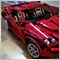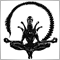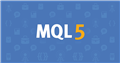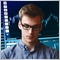# Currency Strength Formula58

Hello Guys

I need help in creating the Currency strength formula that works on comparing the correlation of 8 major pairs that looks like below

50 is neutral, below 40 is weak currency, above 60 is strong currency

Anyone can help on giving the formula on how to calculate those? thanks

Files:543

Play with the RSI1843

energyliger:

I need help in creating the Currency strength formula that works on comparing the correlation of 8 major pairs that looks like below

50 is neutral, below 40 is weak currency, above 60 is strong currency

Anyone can help on giving the formula on how to calculate those? thanks

I found this mq4 file some time back, it contains the formula you want.

Files:
CCFp.mq4  17 kb724Simeon Semenych
• www.mql5.com
Cluster indicators are sets of indicators that divide currency pairs into separate currencies. Indicators allow to trace the relative currency fluctuation, determine the potential of forming new currency trends, receive trade signals and follow medium-term and long-term positions. Theoretical Basis of Building Cluster Indicators...51

Did you find the solution?58

James D. Hunt:
Did you find the solution?

Not yet bro. maybe u got any solutions on how to create the CSR? The requirement is as above58

Seng Joo Thio:

I found this mq4 file some time back, it contains the formula you want.

Thanks man25080

Don't double post! Don't triple post! You already had this thread open.
General rules and best pratices of the Forum. - General - MQL5 programming forum58

Im looking forward to know the formula to create currency strength like the photo in the attachment below. Wondering if any of the FX geniuous know what is the formula, so i can code it on MT4 and MT5

Here are the features

1. It works by comparing movement between 8 major currencies

2. When login to your MT account, you need to download all the 8 major pairs and the chart first so the numbers shown on the indicator would be accurate (it reads the data from 8 major currencies, hence need to download the chart first after all)

3. The numbers are from 0 to 100 (below 40 is considered weak, above 60 is considered strong)

4. The numbers shown in the currency strength (0 to 100) changes every second on all timeframes. Be it on M1, M5, M15, H1, H4 or D1, the currency strength changes every second (my assumption means that it reads the data based on current timeframe and note that the current price always change every second, hence the Currency strength amount changes every second

5. It has 2 decimal places

6. When you open the .ex4 file, there are 2 inputs:
- Number of bars = 90
- Moving Average Periods to Smooth Lines = 0

Those are the standard inputs

7. Number of bars = 90 does NOT refer to 90 candlesticks. It refers to the number of bars used to show the curves, so it does not matter whether the input is 90, 900 or 9000. 90 is enough

8. MA period = 0 means that the 8 curves (refer to the 8 curves of the major pairs) will not be processed by moving averages

Based on these data, can any FX genious helps to create the formula used to create the currency strength?

Thanks a million. Lets post the results here so all of us, FX traders all around the world can learn together

Files:58

Giovanni Riccobene:
Play with the RSI

You mean use RSI formula or modify it to suit the correlation?56

Foluso Makinde:
This is the same photo I am looking for its formula. Did you find the solution? I will be glad if you share with me. Thanks